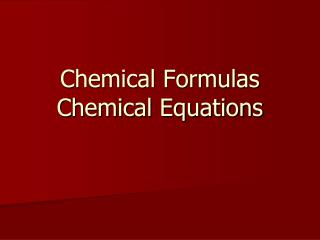DownloadDownload PresentationChemical Formulas Chemical Equations

# Chemical Formulas Chemical Equations

Télécharger la présentation## Chemical Formulas Chemical Equations

- - - - - - - - - - - - - - - - - - - - - - - - - - - E N D - - - - - - - - - - - - - - - - - - - - - - - - - - -
##### Presentation Transcript

1. Chemical FormulasChemical Equations

2. Chemical Formulas • Chemical Formulas are used as a short hand way of describing the atoms that make up a molecule or compound • Chemical Formulas show two things: • Types of atoms (element symbol) • Number of each type of atom in a compound (subscript)

3. CCl4 NaBr Al2O3 Mg(OH)2 Ca(NO3)2 Ca3(PO4)2 Determine the number of each type of atom in the following compounds…

4. Chemical Equations • Chemical Equations are used to show what is present before and after a chemical reaction • Remember, a chemical reaction is any process where a chemical change occurs (and a new substance is produced) • How do you know if a chemical change has occurred?

5. Chemical Equations • Reactants → Products • The substances that undergo the change are referred to as reactants • The substances formed during the reaction are called products

6. Conservation of Mass • During chemical reactions, the mass of the products must ALWAYS equal the mass of the reactants • Mass is never created or destroyed during a chemical reaction – it only changes forms • This is referred to as Conservation of Mass

7. Balancing Equations • Because mass is conserved, the number of each kind of atom must be the same in the reactants and the products • When the number of each type of element is the same in the reactants and the products, the equation is said to be balanced

8. Balancing Equations • A chemical equation must be balanced by adding coefficients in front of formulas and not by changing the subscripts in the formulas

9. Balancing Equations Practice • N2H4 + O2→ N2 + H2O • N2H4 + O2→ N2 + 2 H2O • Mg + HCl → H2 + MgCl2 • Mg + 2 HCl → H2 + MgCl2 • Cu + O2→ CuO • 2 Cu + O2→ 2 CuO • C2H4 + O2 → CO2 + H2O • C2H4 + 3 O2 → 2 CO2 + 2 H2O